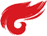函数

# 为什么要用函数

1.在写代码的时候，常常会重复写一段代码，这段代码所执行的功能操作是一样的，只是针对的数据不一样，如果将这段功能写成一个函数模块，在需要用到的时候调用即可，避免了重复写代码的问题。
2.方便代码的维护，当我们知道一段代码出错后，可以逐步检查出某个函数的问题，只需要修改那一个函数即可解决问题。

void bubble(int buf[],int ilen)
{
int i,j,temp;
for(i=0;i<ilen;i++)
{
for(j=0;j<ilen-i-1;j++)
{
if(buf[j-1]>buf[j])
{
temp=buf[j];
buf[j]=buf[j-1];
buf[j-1]=temp;}
}
}
}
}
void bubble(int buf[],int ilen);

# include <stdio.h>

void main()
{
int i;
int chr={27,84,29,19,48,39};
bubble(chr,6);
for (i=0;i<6;i++)
{
printf("%d",chr[i]);
}
printf("\n");
}

# include

using namespace std;

{
return x + y;
}

int add(double x, double y, double z)
{
return x + y + z;
}

int main()
{
cout << add(1, 2) << endl;
cout << add(1.2, 2.2) << endl;
cout << add(1.3, 2.3, 3.3) << endl;
return 0;
}

# 什么是值传递和地址传递

### 值传递

void swap1(int x, int y)
{
int tmp=x;
x=y;
y=tmp;
print(“x=%d, y=%d\n”, x, y);
}

void main()
{
int a=2,b=7;
swap1(a,b) ;
printf(“a=%d, b=%d\n”, a, b);
}

### 地址传递

void swap2(int *px, int py)
{
int tmp=
px;
px=py;
py=tmp;
print(“
px=%d, *py=%d\n”, *px, *py);
}

void main()
{
int a=2;
int b=7;
swap2(&a,&b);
Print(“a=%d, b=%d\n”, a, b);
}

# include<stdio.h>

int Fib(int n){
if(n<0)
return-1;
else if(n0)
return 0;
else if(n
1)
return 1;
else
return Fib(n-1)+Fib(n-2);
}
void main(){
int num;
printf("请输入要求取的第n项斐波那契数列n=");
if(scanf("%d",&num)){
if(num>=0){
printf("%d",Fib(num));
}
else
printf("Error！！！");
return 0;
}
}

posted on 2019-09-16 20:30  neonman  阅读(142)  评论(0编辑  收藏  举报